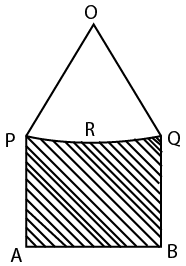Question 100

# In the given figure, PA=QB and PRQ is the arc of the circle, centre of which is O such that angle POQ = 90°. If AB= 25$$\sqrt{2}$$cm and the perpendicular distance of AB from centre O is 30cm. Find the area of the shaded region?Solution

PA = QB and A=B = $$90^{\circ\ }$$

=> PQBA is a rectangle.

Area of the shaded region = (Area of PQBA - Area of the segment PRQ)

$$PQ=AB=25\sqrt{\ 5}$$

$$PO=QO=\frac{PQ}{\sqrt{\ 2}}=25$$ = radius of the circle.

The perpendicular from O to PQ is $$\frac{25}{\sqrt{\ 2}}$$

Distance from the foot of the perpendicular to AB = $$30-\frac{25}{\sqrt{\ 2}}$$

Area of PQBA = $$\left(30-\frac{25}{\sqrt{\ 2}}\right)\left(25\sqrt{\ 2}\right)=750\sqrt{\ 2}-625$$

Area of segment PRQ = Area of sector OPRQ - Area of triangle OPQ = $$\frac{90}{360}\pi\ 25^2-\frac{1}{2}25^2$$

Area of shaded region = $$\left(750\sqrt{\ 2}-625\right)-\left(\frac{90}{360}\pi\ 25^2-\frac{1}{2}25^2\right)$$

= option C